## 5.6Under-relaxation

Sec. 5.5 concludes that the matrix of a typical transport equation is not guaranteed to be diagonally dominant. Some action may therefore be required to ensure a convergent solution.

Under-relaxation is a general method used to improve solution convergence by limiting the amount a variable changes during a solution step.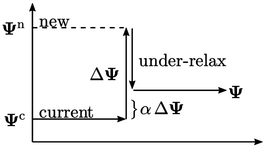During a solution step, assume a single value of a ﬁeld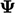in one cell changes from its current value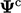to the new value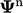. Under-relaxation would limit the change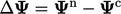by a fraction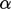,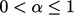, so that the value taken from that solution step is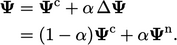(5.13)
In some situations, Eq. (5.13 ) is applied after a solution step. This simple approach is known as ﬁeld under-relaxation, which has one notable disadvantage that it requires additional storage of the intermediate ﬁeld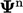in computer memory.

When a solution step involves solving a matrix equation, the new valuescome from an iterative method like Gauss-Seidel. Combining the under-relaxation of Eq. (5.13 ) with the Gauss-Seidel calculation of Eq. (5.4 ) gives: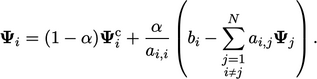(5.14)
Rearranging Eq. (5.14 ) gives the following relation: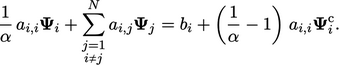(5.15)
Equation 5.15 is simply the matrix equation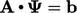modiﬁed by:
• increasing the diagonal coeﬃcients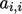by division by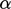;
• multiplying the diﬀerence between the new and original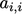coeﬃcients by the current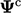and adding it to the source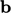.

Modifying the matrix equation this way, known as equation under-relaxation, provides an alternative to Eq. (5.13 ) for under-relaxing a solution of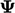, without the temporary storage of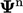.

### Ensuring diagonal dominance

The modiﬁcation toexpressed by Eq. (5.15 ) inspires a strategy to ensure diagonal dominance of the matrix as follows.

Each diagonal coeﬃcient which does not satisfy Eq. (5.9 ) is increased until it is diagonally equal. The change to the coeﬃcient is multiplied by the currentand added to.

This approach to ensure diagonal dominance is eﬀective since it only modiﬁes matrix coeﬃcients where necessary. Otherwise, if the discretisation schemes, and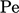and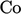are favourable, then no changes to the matrix are necessary.

Notes on CFD: General Principles - 5.6 Under-relaxation